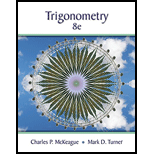Chapter 5, Problem 4GPTrigonometry (MindTap Course List)

8th Edition
Charles P. McKeague + 1 other
ISBN: 9781305652224

Solutions

Chapter
SectionTrigonometry (MindTap Course List)

8th Edition
Charles P. McKeague + 1 other
ISBN: 9781305652224
Textbook Problem

Write y = sin x + cos x in the form y = A sin ( B x + C ) . First identify the values of a, b, and B. Then solve for A and C using the results from Question 3. How does this equation compare with the one you found in Question 1?

To determine

To evaluate:

The values of a, b and B then solve for A andC.

Explanation

Given information:

The equation is given as, sinx+cosx=Asin(Bx+C).

Calculation:

The equation is given as,

sinx+cosx=Asin(Bx+C).

Compare sinx+cosx with asinBx+bcosBx.

Then a=1,b=1 and B=1.

since

a=AcosC.......(1)

b=AsinC.......(2) .

Substitute 1 for a in equation 1 and equation 2 and divide bothe the equation.

11=AcosCAsinCcotC=1C=cot11C=π4,3π4

Substitute π4 for C and 1 for a in equation 1

Still sussing out bartleby?

Check out a sample textbook solution.

See a sample solution

The Solution to Your Study Problems

Bartleby provides explanations to thousands of textbook problems written by our experts, many with advanced degrees!

Get Started

Differentiate the function. y = x5/3 x2/3

Single Variable Calculus: Early Transcendentals, Volume I

Find the mean for the scores in the following frequency distribution table: X f 6 1 5 4 4 2 3 2 2 1

Essentials of Statistics for The Behavioral Sciences (MindTap Course List)

True or False: f(x)f(a)xa may be interpreted as the instantaneous velocity of a particle at time a.

Study Guide for Stewart's Single Variable Calculus: Early Transcendentals, 8th